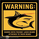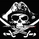16111 views
RSX is a "noise free" version of RSI , with no added lag. This is a port of a clone to Pine.

Use this indicator just like RSI . I have set the default length to 14, feel free to experiment. You can reduce length with out worrying about jaggedness.

Options page has all the configurable parameters. You can use the plot names to setup alerts.

I have couple of more indicators built using this, will post them later. Let me know what you think about this indicator.

RSX Clone compared to normal RSI:

Smoothness helps a lot in identifying the divergences correctly:

http://www.jurikres.com/down__/product_g...

List of my public indicators: http://bit.ly/1LQaPK8
List of my app-store indicators: http://blog.tradingview.com/?p=970
```//
// @author LazyBear
//
// List of my public indicators: http://bit.ly/1LQaPK8
// List of my app-store indicators: http://blog.tradingview.com/?p=970
//
//
study(title="JMA RSX Clone [LazyBear]", shorttitle="RSXC_LB", overlay=false)
src=input(close)
length=input(14)
lvlob = input(70, title="OB Level")
lvlos = input(30, title="OS Level")
mid  = input(50, title="Mid Level")
clampmax = 100
clampmin = 0
f90_ = (nz(f90_) == 0.0) ? 1.0 : (nz(f88) <= nz(f90_)) ? nz(f88)+1 : nz(f90_)+1
f88 = (nz(f90_) == 0.0) and (length-1 >= 5) ? length-1.0 : 5.0
f8 =  100.0*(src)
f18 = 3.0 / (length + 2.0)
f20 = 1.0 - f18
f10 = nz(f8)
v8 = f8 - f10
f28 = f20 * nz(f28) + f18 * v8
f30 = f18 * f28 + f20 * nz(f30)
vC = f28 * 1.5 - f30 * 0.5
f38 = f20 * nz(f38) + f18 * vC
f40 = f18 * f38 + f20 * nz(f40)
v10 = f38 * 1.5 - f40 * 0.5
f48 = f20 * nz(f48) + f18 * v10
f50 = f18 * f48 + f20 * nz(f50)
v14 = f48 * 1.5 - f50 * 0.5
f58 = f20 * nz(f58) + f18 * abs(v8)
f60 = f18 * f58 + f20 * nz(f60)
v18 = f58 * 1.5 - f60 * 0.5
f68 = f20 * nz(f68) + f18 * v18
f70 = f18 * f68 + f20 * nz(f70)
v1C = f68 * 1.5 - f70 * 0.5
f78 = f20 * nz(f78) + f18 * v1C
f80 = f18 * f78 + f20 * nz(f80)
v20 = f78 * 1.5 - f80 * 0.5
f0 = ((f88 >= f90_) and (f8 != f10)) ? 1.0  : 0.0
f90 = ((f88 == f90_) and (f0 == 0.0))  ? 0.0  : f90_
v4_ = ((f88 < f90) and (v20 > 0.0000000001)) ? (v14 / v20 + 1.0) * 50.0 : 50.0
rsx = (v4_ > 100.0) ? 100.0 : (v4_ < 0.0) ? 0.0 : v4_
hline(lvlob, color=red, title="OB Level"), hline(mid,linewidth=2, title="Mid Level"), hline(lvlos, color=green, title="OS Level"),
plot(rsx, color=red, linewidth=2, title="RSXC")
```
List of my free indicators: http://bit.ly/1LQaPK8
List of my indicators at Appstore: http://blog.tradingview.com/?p=970Hello, I am interested in LB_RSRL but cant find anything similar in your list of indicators. Would PM code, please? Thanks